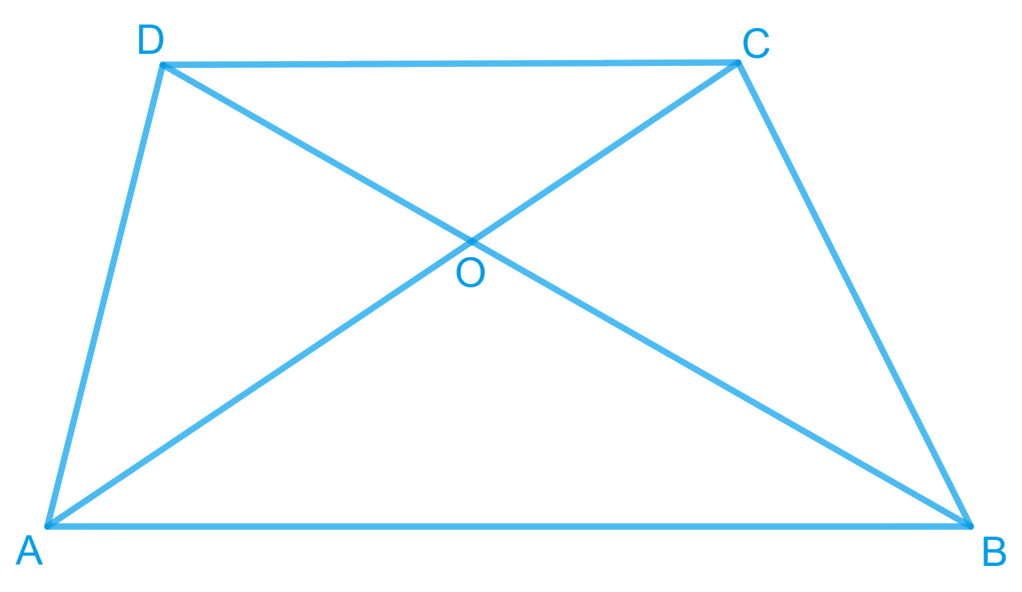# Ex.9.3 Q15 Areas of Parallelograms and Triangles Solution - NCERT Maths Class 9

Go back to  'Ex.9.3'

## Question

Diagonals $$AC$$ and $$BD$$ of a quadrilateral $$ABCD$$ intersect at $$O$$ in such a way that $$ar (AOD) = ar (BOC)$$. Prove that $$ABCD$$ is a trapezium.

Video Solution
Areas Of Parallelograms And Triangles
Ex 9.3 | Question 15

## Text Solution

What is known?

Diagonals $$AC$$ and $$BD$$ of a quadrilateral $$ABCD$$ intersect at $$O$$ in such a way that $$ar (AOD) = ar (BOC).$$

What is unknown?

How we can prove that $$ABCD$$ is a trapezium.

Reasoning:

We can add area of triangle $$AOB$$ on both sides in the given equal area’s triangle. Now we will get two new triangles of equal areas with common base. Now we can use theorem that if Two triangles having the same base (or equal bases) and equal areas lie between the same parallels.

Steps:It is given that,

\begin{align} {\text { Area }(\Delta AOD)}&={\text { Area }(\Delta BOC)} \end{align}

Area $$(\Delta \,AOD)+$$  Area $$(\Delta \,AOB)=$$ Area $$(\Delta \, BOC)+$$ Area $$(\Delta \,AOD)$$

Adding Area $$(\Delta \, AOB )$$  on both the sides

\begin{align} {\therefore \text { Area }(\Delta ADB)}&={\text { Area }(\Delta ACB)}\end{align}

We know that triangles on the same base having areas equal to each other lie between the same parallels.

Therefore, these triangles, \begin{align} (\Delta ADB)\, {\rm{and}} \,(\Delta ACB)\end{align}, are lying between the same parallels. i.e., $$AB \;|| \;CD$$

According to Theorem $$9.3 :$$ Two triangles having the same base (or equal bases) and equal areas lie between the same parallels.

Therefore, $$ABCD$$ is a trapezium.

Video Solution
Areas Of Parallelograms And Triangles
Ex 9.3 | Question 15